# Arc

What area of a circle occupied the flowers planted in the arc of a circle with radius 4 m with central angle 45°?

Correct result:

S =  6.28 m2

#### Solution: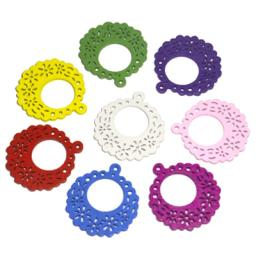We would be pleased if you find an error in the word problem, spelling mistakes, or inaccuracies and send it to us. Thank you!Tips to related online calculators
Check out our ratio calculator.

#### You need to know the following knowledge to solve this word math problem:

We encourage you to watch this tutorial video on this math problem:

## Next similar math problems:

• Divisible by 5How many three-digit odd numbers divisible by 5, which are in place ten's number 3?Convert magnitude of the angle α = 136°18'10" to radians:
• Waiting roomIn the waiting room are people and flies. Together they have 15 heads and 50 legs (fly has 6 legs). How many people and flies are in the waiting room?
• SlopeFind the slope of the line: x=t and y=1+t.If Petra read 10 pages per day, she would read the book two days earlier than she read 6 pages a day. How many pages does a book have?
• CandyPeter had a sachet of candy. He wanted to share with his friends. If he gave them 30 candies, he would have 62 candies. If he gave them 40 candies, he would miss 8 candies. How many friends did Peter have?
• Two numbersFind two numbers whose difference and ratio is 2.
• CancerOf the 80 people 50 people ill cancer. What percentage of people isn't ill?
• EnrollmentThe enrollment at a local college increased 4% over last year's enrollment of 8548. Find the increase in enrollment (x1) and the current enrollment (x2).
• GardenThe garden around the new majer is divided as follows: 35% vegetable, 30% fruit orchards, 10% flowers and the remaining 120 m2 are lawns. What is the total area of the garden?
• Pipe cross sectionThe pipe has an outside diameter 1100 mm and the pipe wall is 100 mm thick. Calculate the cross section of this pipe.
• FlowerbedIn the park there is a large circular flowerbed with a diameter of 12 m. Jakub circulated him ten times and the smaller Vojtoseven times. How many meters each went by and how many meters did Jakub run more than Vojta?
• Bridge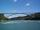The bridge arc has a span 34 m and height 3 m. Calculate the radius of the circle arc of this bridge.
• Type of triangle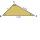How do I find the triangle type if the angle ratio is 2:3:7 ?
• Circumference - a simple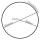What is the ratio of the circumference of any circle and its diameter? Write the result as a real number rounded to 2 decimal places.
• Wheels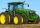Small tractor wheel with a diameter of 60 cm must be rotated 8 times to overcome some pathway. How many times must turn the big tractor wheel with a radius 60 cm to overcome the same distance?
• Arc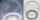The length of the circle is 41 amd arc length of the circle 9. What is the magnitude of the angle of this arc?